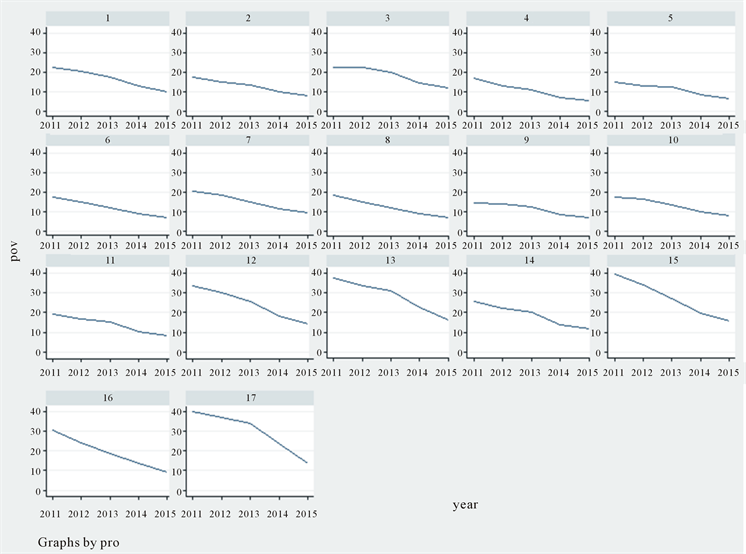# 安徽省第一产业减贫效应分析—基于17个贫困县面板数据Poverty Reduction Effect Empirical Analysis of the First Industry in Anhui Province—Based on Panel Data between 17 Poverty County

• 全文下载: PDF(658KB)    PP.169-175   DOI: 10.12677/SA.2018.72021
• 下载量: 438  浏览量: 1,109

This paper focuses on the empirical analysis for the effect of the first industry may act on re-ducing poverty rate in Anhui province. The author uses the Fixed Effects Model and analyzes the first industry’s impact on the region’s poverty rate based on panel data among 2011-2015 about the 17 poverty-stricken counties in Anhui province. The empirical results point out that the first industry for depressing the poverty rate has a very good effect. It also suggests that the first in-dustry of industry in Anhui province also has a certain positive effect for poverty alleviation. So, it can accelerate the pace of agricultural modernization in Anhui, and the first industry modern-ization may have an assistance in the precision of poverty alleviation.

1. 引言

2. 模型介绍

${y}_{it}={{x}^{\prime }}_{it}\beta +{{z}^{\prime }}_{i}\delta +{u}_{i}+{\epsilon }_{it}\text{\hspace{0.17em}}\text{\hspace{0.17em}}\left(i=1,\cdots ,n;t=1,\cdots ,T\right)$ (1)

${\stackrel{¯}{y}}_{i}={{\stackrel{¯}{x}}^{\prime }}_{i}\beta +{{z}^{\prime }}_{i}\delta +{u}_{i}+{\stackrel{¯}{\epsilon }}_{i}$ (2)

${y}_{it}-{\stackrel{¯}{y}}_{i}={\left({x}_{it}-{\stackrel{¯}{x}}_{i}\right)}^{\prime }\beta +\left({\epsilon }_{it}-{\stackrel{¯}{\epsilon }}_{i}\right)$ (3)

${\stackrel{˜}{y}}_{it}={{\stackrel{˜}{x}}^{\prime }}_{it}\beta +{\stackrel{˜}{\epsilon }}_{it}$ (4)

3. 实证分析

3.1. 数据选取

3.2. 数据的描述性统计

3.3. 数据平衡性的检验

3.4. 豪斯曼检验

3.5. 模型结果Table 1. The descriptive statistics of dataFigure 1. Overview of poverty incidence form 2011 to 2015 in 17 provinces

Standard errors in parentheses. *p < 0.1, **p < 0.05, ***p < 0.01.

4. 结论和政策建议

  倪志良, 郝志景. 财政扶贫支出与减贫的实证分析[J]. 山西财经大学报, 2007(4): 19-22.  沈能, 赵增耀. 农业科研投资减贫效应的空间溢出与门槛特征[J]. 中国农村经济, 2012(1): 69-79.  Zaman, K. and Khilji, B.A. (2013) The Relationship between Growth and Poverty in Forecasting Framework: Pakistan’s Future in the Year 2035. Economic Modelling, 30, 468-491. https://doi.org/10.1016/j.econmod.2012.07.021  Khan, M.A., Khan, M.Z., Zaman, K., Hassan, U. and Umar, S. (2014) Global Estimates of Growth-Inequality-Poverty (GIP) Triangle: Evidence from World Bank’s Classification Countries. Quality & Quantity, 48, 2631-2646. https://doi.org/10.1007/s11135-013-9912-7  李逢春, 唐端. 农产品电商为精准扶贫探路[J]. 国家治理, 2015(33): 37-43.  张蓓. 农产品供给侧结构性改革的国际镜鉴[J]. 改革, 2016(5): 123-130.  程蕾. 公共支出减贫效应实证研究[D]: [硕士学位论文]. 北京: 首都经济贸易大学, 2016.